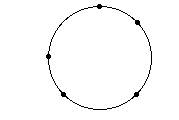## Uniform Circular Motion Activity Sheet

### Purpose:

The purpose of this activity is to explore the characteristics of the motion of an object in a circle at a constant speed.

### Procedure and Questions:

1. Navigate to the Uniform Circular Motion page and experiment with the on-screen buttons in order to gain familiarity with the control of the animation. The object speed, radius of the circle, and object mass can be varied by using the sliders or the buttons. The vector nature of velocity and acceleration can be depicted on the screen. A trace of the objects motion can be turned on, turned off and erased. The acceleration of and the net force acting upon the object are displayed at the bottom of the screen. The animation can be started, paused, continued or rewound.

After gaining familiarity with the program, use it to answer the following questions:

2. Velocity is a vector quantity which has both magnitude and direction. Using complete sentences, describe the body's velocity. Comment on both the magnitude and the direction.

3. TRUE or FALSE?

If an object moves in a circle at a constant speed, its velocity vector will be constant.4. In the diagram at the right, a variety of positions about a circle are shown. Draw the velocity vector at the various positions; direct the v arrows in the proper direction and label them as v. Draw the acceleration vector at the various positions; direct the a arrows in the proper direction and label them as a.

5. Describe the relationship between the direction of the velocity vector and the direction of the acceleration for a body moving in a circle at constant speed.

6. A Puzzling Question to Think About: If an object is in uniform circular motion, then it is accelerating towards the center of the circle; yet the object never gets any closer to the center of the circle. It maintains a circular path at a constant radius from the circle's center. Suggest a reason as to how this can be. How can an object accelerate towards the center without ever getting any closer to the center?

7. A "Thought Experiment": Suppose that an object is moving in a clockwise circle (or at least trying to move in a circle).• Suppose that at point A the object traveled in a straight line at constant speed towards B'. In what direction must a force be applied to force the object back towards B? Draw an arrow on the diagram in the direction of the required force.
• Repeat the above procedure for an object moving from C to D'. In what direction must a force be applied in order for the object to move back to point D along the path of the circle? Draw an arrow on the diagram.
• If the acceleration of the body is towards the center, what is the direction of the unbalanced force? Using a complete sentence, describe the direction of the net force which causes the body to travel in a circle at constant speed.

8. Thinking Mathematically: Explore the quantitative dependencies of the acceleration upon the speed and the radius of curvature. Then answer the following questions.

a. For the same speed, the acceleration of the object varies _____________ (directly, inversely) with the radius of curvature.

b. For the same radius of curvature, the acceleration of the object varies _____________ (directly, inversely) with the speed of the object.

c. As the speed of an object is doubled, the acceleration is __________________ (one-fourth, one-half, two times, four times) the original value.

d. As the speed of an object is tripled, the acceleration is __________________ (one-third, one-ninth, three times, nine times) the original value.

e. As the radius of the circle is doubled, the acceleration is __________________ (one-fourth, one-half, two times, four times) the original value.

f. As the radius of the circle is tripled, the acceleration is __________________ (one-third, one-ninth, three times, nine times) the original value.

### Summary Statement:

Write a conclusion to this lab in which you completely and intelligently describe the characteristics of an object that is traveling in uniform circular motion. Give attention to the quantities speed, velocity, acceleration and net force.

Start Circular Motion Activity.